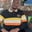Related Tags

swift
literals
communitycreator

# What are literals in Swift?Wale Moshood

A sequence of characters representing constant values stored in variables is referred to as a literal. Literals play a significant role in dealing with values within a program.

Examples of Swift literals are shown below:

"Hi, there!"

3.33333

33

There are different types of Swift literals. They are discussed below:

### 1. String literals

A string literal is a sequence of characters wrapped with a starting double quote and a closing double quote. Special characters are used in string literals to form a branch of string literals known as an escape sequence.

The difference between a string and a character literal is that a string literal contains a sequence of characters, while a character literal contains a single character. An example is shown below:

var sampleCharacter: Character = "S"
print(sampleCharacter)

var sampleString: String = "My name is Sam"
print(sampleString)

### 2. Integer literals

Integer literals are divided into the following:

• Binary literals: It starts with the prefix 0b. It represents binary values.
• Decimal literals: Every value declared in an integer literal is of type decimal. Hence, it is not preceded by any number or character as in the binary literals above.
• Hexadecimal literals: It starts with the prefix 0x. It is used to represent hexadecimal values.
• Octal literals: It starts with the prefix 0o. It is used to represent octal values.

Some examples are shown below:

let binaryInteger = 0b10001
print(binaryInteger)

let decimalInteger = 17
print(decimalInteger)

let octalInteger = 0o21
print(octalInteger)

print(hexadecimalInteger)

### 3. Floating point literals

Floating point literals can either be represented in decimal form or hexadecimal form.

• Decimal floating-point literals: They are made up of a sequence of decimal digits and a decimal fraction or a decimal exponent, or both.
• Hexadecimal floating-point literals: It comprises a 0x prefix, an optional hexadecimal fraction, and a hexadecimal exponent.

An example is shown below:

let sampleDecimalFloat = 3.14e2
print(sampleDecimalFloat)

print(sampleHexadecimalFloat)

### 4. Boolean literals

There are two types of boolean literals. They are:

• true
• false

An example is shown below:

let sample1: Bool = true
print(sample1)

let sample2: Bool = false
print(sample2)

### Output

#### false

RELATED TAGS

swift
literals
communitycreator

CONTRIBUTORWale Moshood
RELATED COURSES

View all Courses

Keep Exploring

Learn in-demand tech skills in half the time